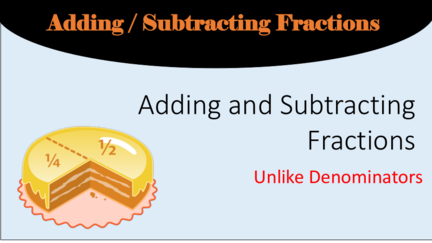# Adding and Subtracting Fractions Unlike DenominatorsContributed by:Add & subtract, unlike fractions. These grade 7 worksheets provide practice in adding and subtracting fractions with both like and unlike denominators.
Fractions
Unlike Denominators
What are Equivalent Fractions?
Fractions that have the same value.
For example, 1/2 = 2/4 = 3/6
EQUIVALENT means EQUAL so all equivalent fractions have to be equal.
I have a pizza and cut in half. I give half to my friend, and I keep half. I take the half I have and cut
it in half again. I still have half of the pizza.
How do you make an equivalent fraction?
MULTIPLY or DIVIDE a fraction by another fraction that is equal to one. In other
words, MULTIPLY or DIVIDE the numerator and denominator by the same number !
For example:
RULE:
subtract fractions unless the
denominators are the same.
denominators!
Fractions with Uncommon
Denominators
STEP 1: ½+¼
You can multiply 2 and 4 to get 8,
Find a common which is a common denominator. Or
denominator. you can list the multiples of 2 and 4
You can either multiply until you find the LCM.
the denominators OR 2 – 2, 4, 6, . . .
find the LCM by listing 4 – 4, 8, 12 . . .
all the multiples of both
numbers. The LCM is 4.
STEP 2:
Write equivalent • ½ = 4/8
fractions with the • ¼ = 2/8
common
denominator.